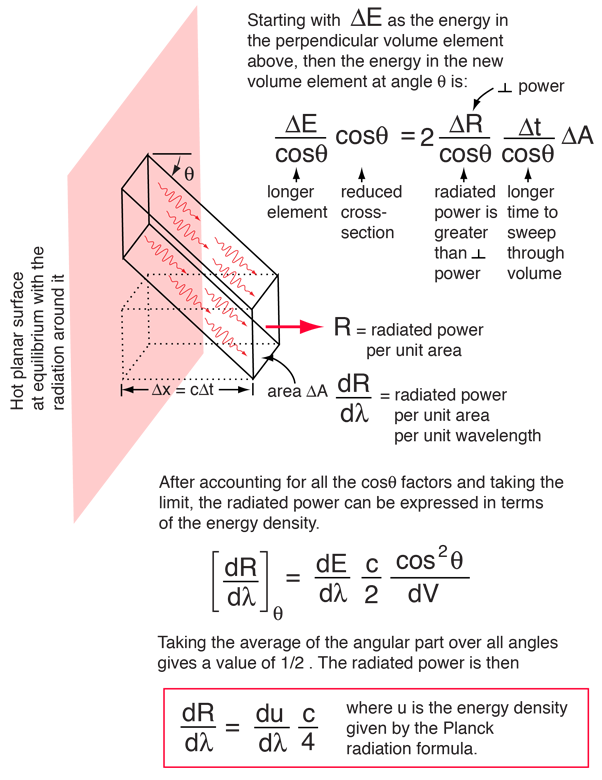# Radiated Energy as a Function of Energy Density

If we consider energy radiated perpendicular to a small increment of area, then it must be noted that half of the energy density in the waves is going toward the walls and half is coming out if the system is in thermal equilibrium. Evaluating the power seen at a given observation point requires a consideration of the geometry:Other elements of area on the surface send radiation through the specified surface area, and that radiation has a component perpendicular to the surface. To evaluate that contribution, consider a surface element at an arbitrary angle as shown below.The factor c/4 developed here involves an integral over all angles. It is important because it relates the radiated energy to the energy density given by the Planck radiation formula.

### Development of the Rayleigh-Jeans Law

Index

Rayleigh- Jeans references